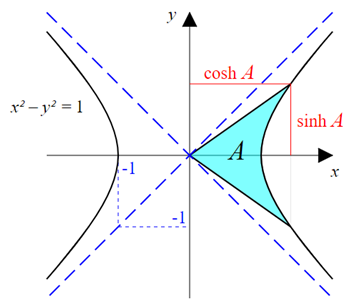Excel math hyperbolic functions: sinh, cosh, tanh, csch, sech, coth

Excel Function HYPERBOLIC FUNCTIONS

A hyperbolic function is a one which specifies the relationship between two distances; one from origin to a

point on the hyperbola and other from coordinate axes to the same point on the hyperbola.

The main difference between normal trigonometric functions and hyperbolic functions is that, the hyperbolic functions

form half a circle instead of full circle.Excel Function SINH

The SINH() function is used to determine the hyperbolic sine value of the number specified.

This function takes single argument which is the angle expressed as number.

The angle is always in radian

If you want to use degree transform the angle before with =RADIANS(angle in degree)

 Angle(deg) Angle (Rad) Formula SINH() 0 0 =SINH(M10) 0 30 0.523598775598299 =SINH(M11) 0.54785347388804 90 1.5707963267949 =SINH(M12) 2.30129890230729 180 3.14159265358979 =SINH(M13) 11.5487393572577 360 6.28318530717959 =SINH(M14) 267.744894041016

Excel Function COSH

The COSH() function is used to determine the hyperbolic cosine value of the number specified.

This function takes single argument which is the angle expressed as number.

The angle is always in radian

If you want to use degree transform the angle before with =RADIANS(angle in degree)

 Angle(deg) Angle (Rad) Formula COSH() 0 0 =COSH(M23) 1 30 0.523598775598299 =COSH(M24) 1.14023832107643 90 1.5707963267949 =COSH(M25) 2.50917847865806 180 3.14159265358979 =COSH(M26) 11.5919532755215 360 6.28318530717959 =COSH(M27) 267.746761483748

Excel Function TANH()

The TANH() function is used to determine the hyperbolic tangent value of the number specified.

This function takes single argument which is the angle expressed as number.

The angle is always in radian

If you want to use degree transform the angle before with =RADIANS(angle in degree)

 Angle(deg) Angle (Rad) Formula TANH() 0 0 =TANH(M36) 0 30 0.523598775598299 =TANH(M37) 0.480472778156452 90 1.5707963267949 =TANH(M38) 0.917152335667274 180 3.14159265358979 =TANH(M39) 0.99627207622075 360 6.28318530717959 =TANH(M40) 0.999993025339611

Excel Function CSCH()

The CSCH() function is used to determine the hyperbolic cosine of the number specified.

This function takes single argument which is the angle expressed as number.

The angle is always in radian

If you want to use degree transform the angle before with =RADIANS(angle in degree)

 Angle(deg) Angle (Rad) Formula CSCH() 10 0.174532925199433 =CSCH(M49) 5.70059217731439 30 0.523598775598299 =CSCH(M50) 1.82530557468795 90 1.5707963267949 =CSCH(M51) 0.434537208094696 180 3.14159265358979 =CSCH(M52) 8.65895375300469E-02 360 6.28318530717959 =CSCH(M53) 3.73489848828567E-03

Excel Function SECH

The SECH() function is used to determine the hyperbolic secant of the number specified.

This function takes single argument which is the angle expressed as number.

The angle is always in radian

If you want to use degree transform the angle before with =RADIANS(angle in degree)

 Angle(deg) Angle (Rad) Formula SECH() 10 0.174532925199433 =SECH(M62) 0.984960079661621 30 0.523598775598299 =SECH(M63) 0.877009640454779 90 1.5707963267949 =SECH(M64) 0.398536815338387 180 3.14159265358979 =SECH(M65) 8.62667383340544E-02 360 6.28318530717959 =SECH(M66) 3.73487243863713E-03

Excel Function COTH

The COTH() function is used to determine the hyperbolic cotangent of the number specified.

This function takes single argument which is the angle expressed as number.

The angle is always in radian

If you want to use degree transform the angle before with =RADIANS(angle in degree)

 Angle(deg) Angle (Rad) Formula COTH() 10 0.174532925199433 =COTH(M75) 5.78763778860236 30 0.523598775598299 =COTH(M76) 2.08128336393364 90 1.5707963267949 =COTH(M77) 1.09033141072737 180 3.14159265358979 =COTH(M78) 1.00374187319732 360 6.28318530717959 =COTH(M79) 1.00000697470904